# microPop Tutorial

library(microPop)
#> Loading required package: visNetwork

# Overview

MicroPop is an R package which simulates/predicts the growth of interacting populations of microbiota.

It is described in the paper:

Kettle H, G Holtrop, P Louis, HJ Flint. 2018. microPop: Modelling microbial populations and communities in R. Methods in Ecology and Evolution, 9(2), p399-409. doi: 10.1111/2041-210X.12873 https://besjournals.onlinelibrary.wiley.com/doi/full/10.1111/2041-210X.12873

The user specifies the system via a number of input files (csv files that become dataframes) and the function microPopModel() will construct and solve the necessary equations (ordinary differential equations) and provide an output containing the solution (e.g. the concentrations of microbes, substrates and metabolites at the required time points) as well as all the settings/parameters involved in the simulation, and plots of the microbes and resources over time.

## This Tutorial

A zip file with all the files used in this tutorial is given here:

https://www.bioss.ac.uk/people/helen/microPop/microPopTutorial.zip

Or see the webpage: https://www.bioss.ac.uk/people/helen/microPop/microPop.html

# Getting started

library(microPop)
#check package version
packageVersion("microPop")
#>  '1.6'

Make sure you are using version 1.6 or above.

We begin by making a csv file for our microbe ('MFG1.csv'; file provided in the InputFiles folder) and then loading it into R using the microPop function 'createDF()'. Note, it is better to use createDF() than the base R functions as no need to specify stringsAsFactors=FALSE etc.

M1=createDF(file='MFG1.csv')

M1 looks like this:

units S1 P1
Rtype none S P
halfSat g/l 0.001 NA
yield g/g 0.3 NA
maxGrowthRate /d 5 NA
stoichiom mol 1 2
keyResource none NA
pHcorners pH
numPathways none 1

Where

• Rtype is the type of resource (S=substrate, P=product). Since products are often also substrates (e.g. in cross feeding) we use 'resources' to refer to both.

• keyResource is only important for specific cases (more later)

• pHcorners is only important if you want pH to limit growth (more later)

• numPathways is only important if the microbe has more than one metabolic pathway

• halfSat and maxGrowthRate are shown in plot below. The halfSat is the half saturation constant i.e. the amount of substrate needed to give half the maximum growth (assuming Monod Equation growth).

• The yield is g M1/g S1 i.e. the grams of substrate needed per gram of microbial growth.

• The units column is not used by microPop but is there for your reference.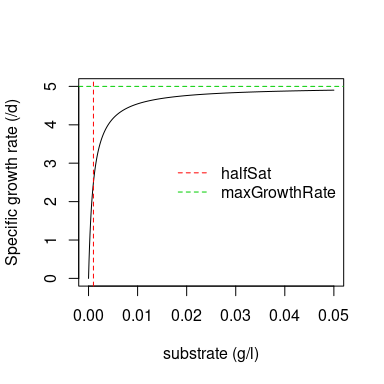Now we have specified the microbe we need to tell microPop about the environment it is in. We do this using 'system information (SI) files'. We can make these files in excel (or any editor) and save them as csv and read into R using createDF() again:

res.SI=createDF(file='resourceSI.csv')

res.SI looks like this:

Units S1 P1
startValue g/l 10 0
inflowRate g/l/d 1 0
washOut /d 1 1
molarMass g/mol 100 50

Note, we have specified the starting value of each resource, the amount that is flowing into the system and the specific wash out rate (e.g. if the system has a turnover time of 2 days then this is 0.5 /d and each resource, R, (g/l) leaves at the rate 0.5*R g/l/d ). For convenience we also specify the molar mass of each resource here.

We now read in the microbial SI file:

mic.SI=createDF(file="microbeSI.csv")

mic.SI looks like:

Units M1
startValue g/l 1
inflowRate g/l/d 0
washOut /d 1

Note, once these input files are defined as objects in our workspace we can edit them any way we like in R.

We now use the input arguments in the microPopModel() function to define the remaining information about the system i.e. which microbes are there (microbeNames) and how long we want to simulate (times) and we tell it which SI data frames to use. Note, here times is a sequence from 0 to 1 going up in 0.001 intervals. There are no units specified but since our input files all have time units of d then this is what microPop assumes are the units for times. You can use any time units you like but you must be consistent throughout all your input information. Similarly only use one mass unit. MicroPop is based on mass balance equations therefore you can not use moles only mass or concentrations for quantities.

out=microPopModel(microbeNames = 'M1',
times=seq(0,1,0.001),
resourceSysInfo=res.SI,
microbeSysInfo=mic.SI,
plotOptions=list(plotFig=FALSE)
)
par(mfrow=c(1,2))
plotMicrobes(out,cex.title=0.7,cex.legend=0.5,cex.ax=0.7)
plotResources(out,cex.title=0.7,cex.legend=0.5,cex.ax=0.7)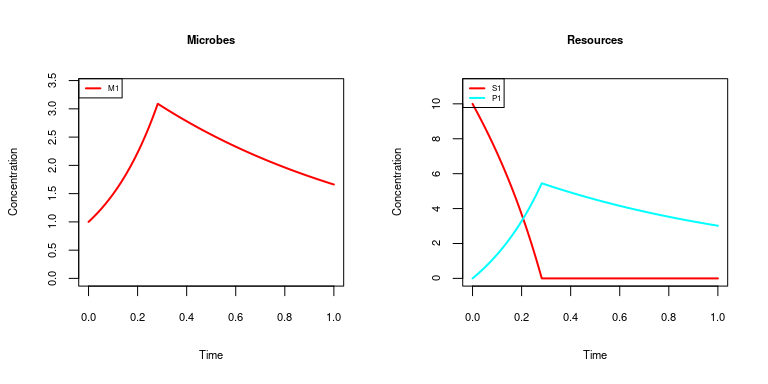When microPop runs it will automatically produce two plots - one for the resources (i.e. substrates and products) and another for the microbes. This does not work well with R markdown (used to contruct this vignette) so we turn this off using plotOptions(plotFig=FALSE) and then call the two plotting functions ( plotMicrobes and plotResources) separately afterwards (note, we will not always show this plotting code in the vignette from now on).

Let's look at the output ('out'):

names(out)
#>  "solution" "parms"

It is a list with 2 elements. The first item, 'solution', is a matrix of the state variables (M1, S1 and P1) at the times specified in 'times'. Let's look at the first few lines:

head(out$solution) #> time M1 S1 P1 pH #> [1,] 0.000 1.000000 10.000000 0.00000000 NA #> [2,] 0.001 1.004008 9.974314 0.01168303 NA #> [3,] 0.002 1.008031 9.948588 0.02340120 NA #> [4,] 0.003 1.012071 9.922820 0.03515467 NA #> [5,] 0.004 1.016127 9.897010 0.04694356 NA #> [6,] 0.005 1.020199 9.871158 0.05876805 NA If you are familiar with R you can make your own plots from the matrix out$solution, e.g.

plot(range(out$solution[,'time']),c(0,10),type='n',xlab='Time (d)',ylab='concentration (g/l)') lines(out$solution[,'time'],out$solution[,'M1'],col='black') lines(out$solution[,'time'],out$solution[,'S1'],col='red') lines(out$solution[,'time'],out$solution[,'P1'],col='blue') legend('topright',c('M1','S1','P1'),col=c('black','red','blue'),lty=1)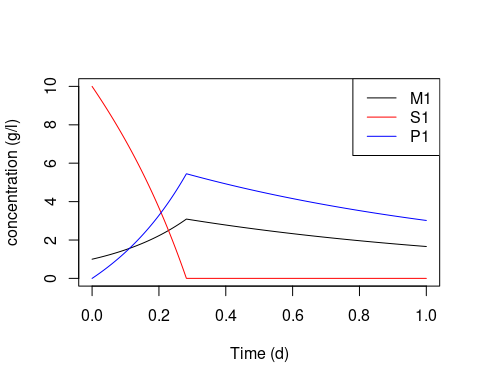The second item, 'parms' is another list containing all the information about the simulation. We can look at the names of its entries: names(out$parms)
#>   "keyRes"               "numPaths"             "allStrainNames"
#>   "resourceNames"        "microbeNames"         "numStrains"
#>   "Pmats"                "Smats"                "strainPHcorners"
#>  "nonBoostFrac"         "pHLimit"              "pHVal"
#>  "molarMass"            "bacCutOff"            "balanceTol"
#>  "checkMassConv"        "times"                "networkAnalysis"
#>  "myPars"               "pHFunc"               "pHLimFunc"
#>  "extraGrowthLimFunc"   "growthLimFunc"        "combineGrowthLimFunc"
#>  "uptakeFunc"           "productionFunc"       "combinePathsFunc"
#>  "massBalanceFunc"      "entryRateFunc"        "removalRateFunc"

You can look at any of these entries to check the model settings for the simulation, e.g.

out$parms$microbeNames
#>  "M1"
out$parms$resourceNames
#>  "S1" "P1"

Let's see what happens if we add another microbe to this system.

The new microbe (M2) has a high maximum growth rate but also a high half-saturation constant compared with M1.

M2=createDF(file="MFG2.csv")

M2 looks like this:

units S1 P1
Rtype none S P
halfSat g/l 1 NA
yield g/g 0.3 NA
maxGrowthRate /d 20 NA
stoichiom mol 1 2
keyResource none NA
pHcorners pH units NA NA
numPathways none 1

We also need to add this microbe to the microbe Sys Info file. Let's assume for simplicity that it has the same conditions as M1, then we can add the M1 column to the dataframe and name it M2:

micNew.SI = cbind(mic.SI,M2=mic.SI[,'M1']) #cbind is a function which binds columns
Units M1 M2
startValue g/l 1 1
inflowRate g/l/d 0 0
washOut /d 1 1

We now run a simulation with the two microbes and extend the time period to 3 days

out=microPopModel(microbeNames = c('M1','M2'),
times=seq(0,3,0.001),
resourceSysInfo=res.SI,
microbeSysInfo=micNew.SI,
plotOptions = list(plotFig=FALSE)
)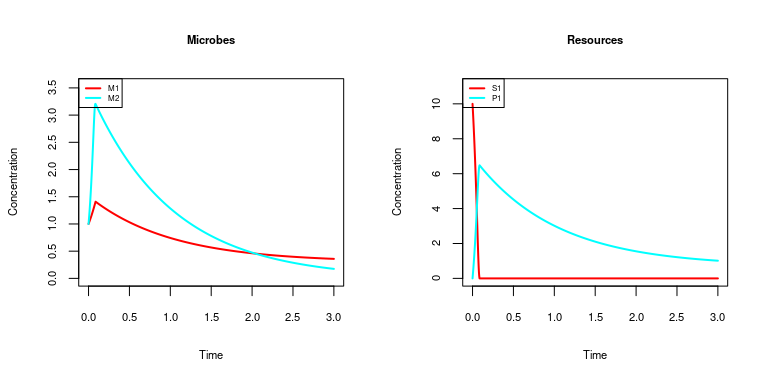## QUESTIONS

A. Why is concentration of M2 > M1 near the beginning but M2 < M1 near the end? [hint: look at parameter values]

#SOLUTION
#look at the final concentrations:

out$solution[nrow(out$solution),c('M1','M2')]
#>        M1        M2
#> 0.3589012 0.1750382

#M1 and M2 have the same yield but M2 has a higher max growth rate and a higher halfSat.
#As time goes on the substrate concentration is low so the max growth rate is not important.
#We change half-sat of M2 to 1e-4 (i.e. less than M1) and look what happens:
newM2=M2 #make a new copy of M2 to play with
newM2['halfSat','S1']=1e-4
micNew.SI = cbind(micNew.SI,newM2=micNew.SI[,'M2']) #add newM2 to the microbe SI data frame (assume same conditions as M2)

out=microPopModel(microbeNames = c('M1','newM2'),
times=seq(0,3,0.001),
resourceSysInfo=res.SI,
microbeSysInfo=micNew.SI,
plotOptions = list(plotFig=FALSE)
)
#>  "Set up completed, ODE solver called..."

out$solution[nrow(out$solution),c('M1','newM2')]
#>         M1      newM2
#> 0.07041673 0.46358173
#Now M2 > M1 near the end of the simulation

B. What do you think will happen to M2 if we run the simulation for much longer e.g. 10 days?

#SOLUTION
out=microPopModel(microbeNames = c('M1','M2'),
times=seq(0,10,0.001),
resourceSysInfo=res.SI,
microbeSysInfo=micNew.SI,
plotOptions = list(plotFig=FALSE)
)
#>  "Set up completed, ODE solver called..."

out$solution[nrow(out$solution),c('M1','M2')]
#>           M1           M2
#> 0.2999732783 0.0001651169
#M2 dies out as it can't compete with M1 at low substrate concentrations.

## EXERCISE

Run microPop with different parameter values for yield, maximum growth and half saturation constant. Which parameters are important in the initial stages and which determine the steady state values (i.e. when the solution has stopped changing in time)?

#SOLUTION
#start by making M1 and M2 the same:
newM2[c('halfSat','yield','maxGrowthRate'),'S1'] = M1[c('halfSat','yield','maxGrowthRate'),'S1']

out=microPopModel(microbeNames = c('M1','newM2'),
times=seq(0,6,0.001),
resourceSysInfo=res.SI,
microbeSysInfo=micNew.SI,
plotOptions=list(plotFig=FALSE)
)
#>  "Set up completed, ODE solver called..."

out$solution[nrow(out$solution),c('M1','newM2','S1')]
#>           M1        newM2           S1
#> 0.1557893117 0.1557893117 0.0002384204

#Now investigate the effect of yield values:

#make M2 have a lower yield than M1
newM2['yield','S1'] = 0.5*as.numeric(M1['yield','S1'])

out=microPopModel(microbeNames = c('M1','newM2'),
times=seq(0,6,0.001),
resourceSysInfo=res.SI,
microbeSysInfo=micNew.SI,
plotOptions = list(plotFig=FALSE)
)
#>  "Set up completed, ODE solver called..."
out$solution[nrow(out$solution),c('M1','newM2','S1')]
#>           M1        newM2           S1
#> 0.1046860252 0.1046860252 0.0002360937

Both microbes have the same steady state concentration i.e. neither microbe out competes the other but both their steady state values are lower than before. However, S1 has the same value as before.

Mathematically the steady state solution for two co-existing microbes shows $V^{out}=G_{M1}\frac{S1}{S1+K1}=G_{M2}\frac{S1}{S1+K2}$ i.e. they both have a specific growth rate equal to the wash out rate.

For $$K1=K2=K$$ and $$G1^{\max}=G2^{\max}=G^{\max}$$, the steady state substrate concentration is

$S=\frac{V^{out} K}{G^{\max}-V^{out}}$ i.e. it is unaffected by yield (as confirmed by our microPop solution).

For $$M1=M2=M$$, the steady state concentrations of each microbe is $M=\frac{\dot{S1^{in}}-V^{out}S1}{G\left(\frac{1}{Y1}+\frac{1}{Y2}\right)}$ i.e. SS value of M depends on the yield values.

We can see from the first equation that for co-existence, the microbes must have identical growth rates. If $$G^{\max}$$ and $$K$$ are not the same for both microbes then this is not the case, therefore, differing values of these parameters lead to exclusion of one microbe. Which microbe survives depends on the relative sizes of S1 and K as if $$S1>>K$$ then $$\frac{S1}{S1+K}$$ approaches 1 (and $$G^{\max}$$ is important), and if $$S1<<K$$ it approaches 0.

# Multiple Strains

When we define the input file for a microbe, this can be viewed as a single strain or as a functional group. If it is a functional group then we can add a number of strains to it. We do this by setting the numStrains argument in microPopModel() to more than 1. We can then specify the parameters, e.g. halfSat, yield, maxGrowthRate etc, for these strains to vary randomly around the average value for the group. This way we have a population of strains with the same metabolic paths but with slightly different growth traits.

out=microPopModel(microbeNames = c('M1','M2'),
times=seq(0,3,0.001),
resourceSysInfo=res.SI,
microbeSysInfo=micNew.SI,
numStrains=3,
strainOptions=list(randomParams=c('halfSat','yield','maxGrowthRate'),
seed=1,distribution='uniform',
percentTraitRange=20,
plotOptions = list(plotFig=FALSE)
)
par(mfrow=c(1,2))
plotMicrobes(out,cex.title=0.7,cex.legend=0.5,cex.ax=0.7,sumOverStrains = FALSE)
plotResources(out,cex.title=0.7,cex.legend=0.5,cex.ax=0.7)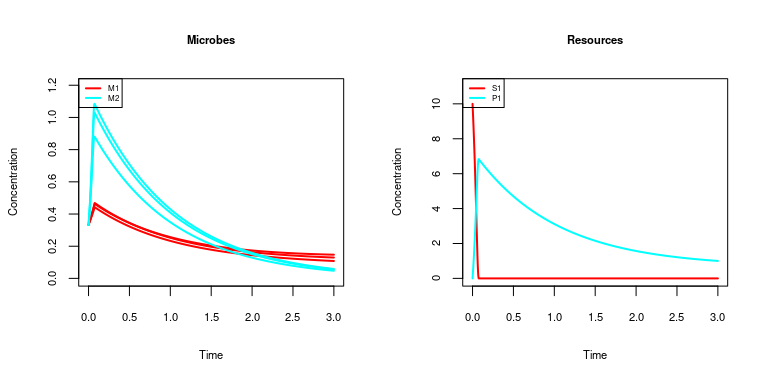Here there are 3 strains in each functional group (M1, M2) whose parameter values vary by +/- 20% from a uniform distribution around the mean value specified in the input data frames. The stochastically-generated values have been picked so there are trade-offs between the maximum growth rate and the half-saturation constant. Note that we have to specify sumOverStrains=FALSE to plot each strain in plotMicrobes and if we were using the plotting function run in _microPopModel we would use plotOptions = list(plotFig=TRUE,sumOverStrains=FALSE).

We can look at the final distribution of biomass:

barplot(out$solution[nrow(out$solution),out$parms$allStrainNames],col=c(rep('red',3),rep('cyan',3)))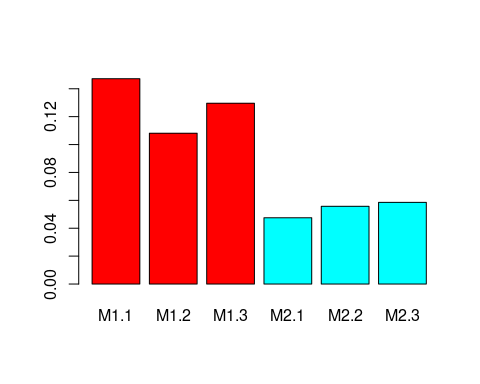Note the microPop nomenclature for naming the stochastic strains and these names are found in

out$parms$allStrainNames
#>  "M1.1" "M1.2" "M1.3" "M2.1" "M2.2" "M2.3"

It is also possible to have different numbers of strains in each group. In this case numStrains is a named vector,

out=microPopModel(microbeNames = c('M1','M2'),
times=seq(0,3,0.001),
resourceSysInfo=res.SI,
microbeSysInfo=micNew.SI,
numStrains=c(M1=2,M2=3),
strainOptions=list(randomParams=c('halfSat','yield','maxGrowthRate'),
seed=1,distribution='uniform',
percentTraitRange=20,
plotOptions = list(plotFig=FALSE)
)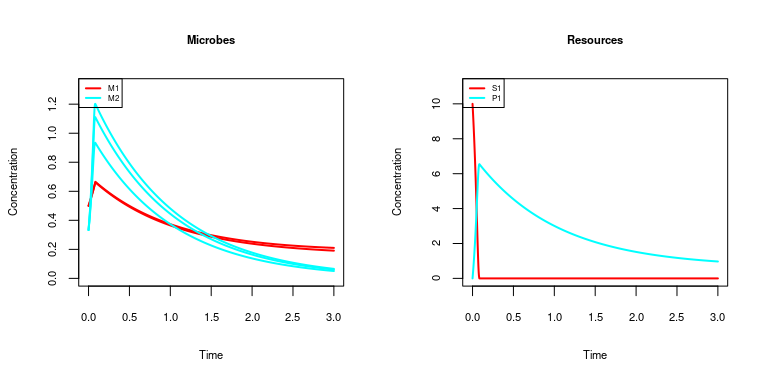## EXERCISE

Try increasing the number of strains (beware that more strains takes longer to compute though!) and change the range and distribution of the trait values.

What happens if you run the simulation for 10 days - do all of the strains survive?

#SOLUTION
out3=out #save the 3 day solution

out10=microPopModel(microbeNames = c('M1','M2'),
times=seq(0,10,0.001),
resourceSysInfo=res.SI,
microbeSysInfo=micNew.SI,
numStrains=3,
strainOptions=list(randomParams=c('halfSat','yield','maxGrowthRate'),
seed=1,distribution='uniform',
percentTraitRange=20,
plotOptions = list(plotFig=FALSE)
)
#>  "Set up completed, ODE solver called..."
barplot(out10$solution[nrow(out10$solution),out10$parms$allStrainNames],col=c(rep('red',3),rep('cyan',3)))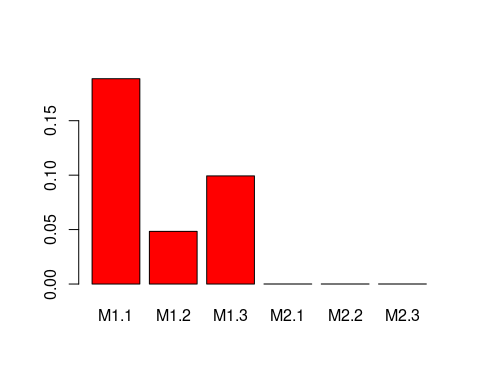out20=microPopModel(microbeNames = c('M1','M2'),
times=seq(0,20,0.001),
resourceSysInfo=res.SI,
microbeSysInfo=micNew.SI,
numStrains=3,
strainOptions=list(randomParams=c('halfSat','yield','maxGrowthRate'),
seed=1,distribution='uniform',
percentTraitRange=20,
plotOptions = list(plotFig=FALSE)
)
#>  "Set up completed, ODE solver called..."
barplot(out20$solution[nrow(out20$solution),out20$parms$allStrainNames],col=c(rep('red',3),rep('cyan',3)))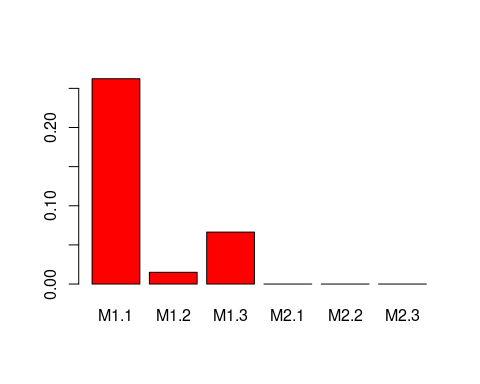#Plot all together
barplot(rbind(out3$solution[nrow(out3$solution),out3$parms$allStrainNames],
out10$solution[nrow(out10$solution),out10$parms$allStrainNames],
out20$solution[nrow(out20$solution),out20$parms$allStrainNames]),
beside=TRUE,legend.text = c('3 days','10 days','20 days'))
#> Warning in rbind(out3$solution[nrow(out3$solution),
#> out3$parms$allStrainNames], : number of columns of result is not a multiple of
#> vector length (arg 1)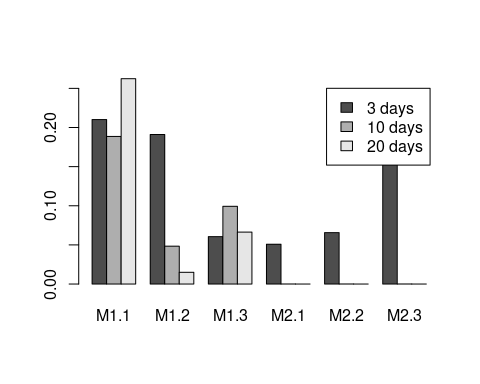It is clear that all strains are decreasing in biomass apart from one.

# Rate Functions

MicroPop uses ordinary differential equations which define the rates of changes of the microbes, substrates and metabolic products (the "state variables") involved in the system. However, the general user does not need to concern themselves with these unless they want to change the default functions.

There are default functions for

• rate of entry of each state variable to the system -- entryRateFunc()

• rate of exit of each state variable -- removalRateFunc()

• the limit on the maximum growth rate e.g. Monod equation -- growthLimFunc()

• how to combine growth on multiple resources -- combineGrowthLimFunc()

• resource uptake rate due to microbial growth -- uptakeFunc()

• production rate of metabolites -- productionFunc()

• pH at each time point -- pHFunc()

• the pH limit on growth -- pHLimFunc()

• other limits on growth (e.g. inhibition) -- extraGrowthLimFunc()

• combining growth on multiple metabolic paths -- combinePathsFunc()

These are all contained in a list called rateFuncs which has a default version called rateFuncsDefault. You can see this if you type rateFuncsDefault at the prompt (it is quite long though!). To just see the elements you can type

  names(rateFuncsDefault)
#>   "pHFunc"               "pHLimFunc"            "extraGrowthLimFunc"
#>   "growthLimFunc"        "combineGrowthLimFunc" "uptakeFunc"
#>   "productionFunc"       "combinePathsFunc"     "massBalanceFunc"
#>  "entryRateFunc"        "removalRateFunc"

To look at one function, e.g. growthLimFunc use

  rateFuncsDefault$growthLimFunc #> function (strainName, groupName, pathName, varName, resourceValues, #> allSubType, strainHalfSat, stateVarValues, parms) #> { #> if (resourceValues[varName] <= 0) { #> v = 0 #> } #> else { #> if (allSubType[varName] == "Sb" | allSubType[varName] == #> "Se") { #> v = resourceValues[varName]/(resourceValues[varName] + #> strainHalfSat[varName]) #> } #> if (allSubType[varName] == "S" | allSubType[varName] == #> "Sm") { #> v = resourceValues[varName]/(strainHalfSat[varName] * #> (1 + sum(resourceValues[allSubType == "S" | allSubType == #> "Sm"]/strainHalfSat[allSubType == "S" | allSubType == #> "Sm"]))) #> } #> } #> return(max(v, 0)) #> } #> <bytecode: 0x77ec158> #> <environment: namespace:microPop> This looks complicated because microPop is very generic so there are different equations for dealing with different types of resource, e.g. substitutable (S) and essential (Se) resources. Also there may be boosting resource (Sb) e.g. Acetate, or for viruses a microbe may be a resource (Sm). However, should you wish to replace this with your own function (which must have the same form of input arguments and output) then this is done as follows:  myRateFuncs = rateFuncsDefault #make your own copy of the rateFuncs list myRateFuncs$growthLimFunc = myGrowthFunction #assign your own function  

where myGrowthFunction is your own function (which you will need to define before this point). Then when you call microPop you use the new rateFuncs list , i.e.

out=microPopModel(microbeNames = 'M1',
times=seq(0,10,0.001),
resourceSysInfo=res.SI,
microbeSysInfo=mic.SI,
rateFuncs=myRateFuncs
)

# pH

It is well established that pH has a significant effect on microbial growth rates. We now import a new microbe, M3, which has pH preferences, consumes 2 substrates and generates 2 products.

M3=createDF(file='MFG3.csv')

M3 looks like this:

units S1 S2 P1 P2
Rtype none S S P P
halfSat g/l 0.001 0.001 NA NA
yield g/g 0.3 0.3 NA NA
maxGrowthRate /d 5 10 NA NA
stoichiom mol 2 2 3 2
keyResource none
pHcorners pH 4 5 7 8
numPathways none 1

The pH preference is defined by pHcorners which describe the following shape:

plot(as.numeric(M3['pHcorners',2:5]),c(0,1,1,0),type='l',xlab='pH',ylab='pH limitation',col='blue',lwd=2)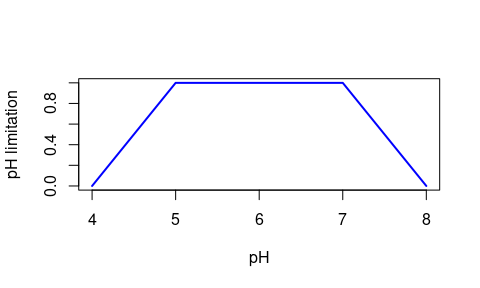This multiplies the maxGrowthRate, thus Where the limitation is 1 there is no restriction on growth and where the limit is 0 there is no growth; inbetween it it linearly scaled.

We also need to read in the new resource SI file with the system information about these new resources (S2,P2).

res.SI2=createDF(file='resourceSI2.csv')

res.SI2:

Units S1 P1 S2 P2
startValue g/l 2 0 2 0
inflowRate g/l/d 5 0 5 0
washOut /d 1 1 1 1
molarMass g/mol 100 50 100 25
mic.SI2=createDF(file='microbeSI2.csv')

mic.SI2

Units M1 M2 M3
startValue g/l 1 1 1
inflowRate g/l/d 0 0 0
washOut /d 1 1 1

In order for the pH preferences to be relevant we need to specify the pH of the system. This can be done using the pHVal argument in microPopModel() (see code below) or if you want pH to change over time then you can use pHFunc in the rateFuncs list. To make sure the pH affects the growth we also need to set the microPopModel argument, pHLimit=TRUE. We now run simulations for different pHs and plot the results

out1=microPopModel(microbeNames = 'M3',
times=seq(0,5,0.001),
resourceSysInfo=res.SI2,
microbeSysInfo=mic.SI2,
pHLimit=TRUE,
pHVal=4.1,
plotOptions=list(plotFig=FALSE)
)

out2=microPopModel(microbeNames = 'M3',
times=seq(0,5,0.001),
resourceSysInfo=res.SI2,
microbeSysInfo=mic.SI2,
pHLimit=TRUE,
pHVal=6,
plotOptions=list(plotFig=FALSE)
)
plot(range(out1$solution[,'time']),c(0,max(c(out1$solution[,'M3'],out2$solution[,'M3']))),type='n',xlab='Time (d)',ylab='Concentration (g/l)', main='M3',lwd=2) lines(out1$solution[,'time'],out1$solution[,'M3'],col='black',lwd=2) lines(out2$solution[,'time'],out2$solution[,'M3'],col='red',lwd=2) legend('right',col=1:2,lty=1,legend=c(4.1,6),title='pH',lwd=2)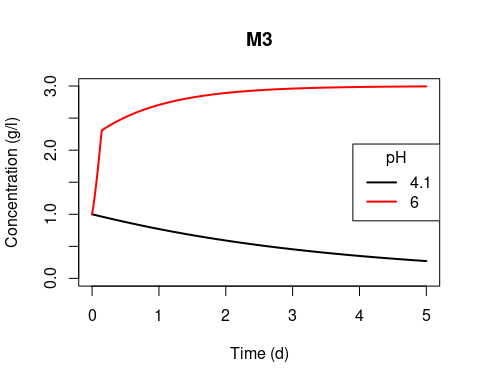## EXERCISE Run a simulation where the pH changes from 6 to 4.1 after day 2. [Hint: you will need to change pHFunc in the rateFuncs list as described above for the growth function] #SOLUTION myRateFuncs=rateFuncsDefault myRateFuncs$pHFunc=function(time,parms){
if (time<=2){
pH=6
}else{
pH=4.1
}
return(pH)
}

out=microPopModel(microbeNames = 'M3',
times=seq(0,5,0.001),
resourceSysInfo=res.SI2,
microbeSysInfo=mic.SI2,
pHLimit=TRUE,
rateFuncs=myRateFuncs
)
#> path1
#>    ""
#>  "MICROPOP NOTE TO USER: The key resource for M3 on path 1 is not used"
#>  "Set up completed, ODE solver called..."

# Included Data and Demo files

The microPop package comes with many microbial data frames and system information files included. These are for modelling the human colonic microbiota, the rumen etc (they are described in Kettle et al, 2018 and used in the demofiles). You can get a list of the included data sets using:

data(package='microPop')

Or on the Environment tab in Rstudio, change the drop down menu from Global Environment to package:microPop and then you will see the data sets in the package. You can click on these to look at them. If you are not in Rstudio you can use View() to look at them or just type the name of the data frame at the R prompt.

There are also a number of demo files included in the package. These correspond to the examples in Kettle et al (2018). To find where they are on your computer, use

system.file('DemoFiles/',package='microPop')

You can then view them in any editor.

To run any of the examples in Kettle et al 2018, you can use the function runMicroPopExample(). E.g.

runMicroPopExample('human1') 

## Demofile: human1.R

This uses microPop to simulate the growth of 3 microbial functional groups that are found in the human colon (see Table 1 of Kettle et al., 2018). Note that we don't need to define any of these data frames as they are already included.

out=microPopModel(
microbeNames=c('Bacteroides','NoButyStarchDeg','Acetogens'),
times=seq(0,4,1/24),
resourceSysInfo=resourceSysInfoHuman,
microbeSysInfo=microbeSysInfoHuman,
plotOptions = list(plotFig=FALSE)
)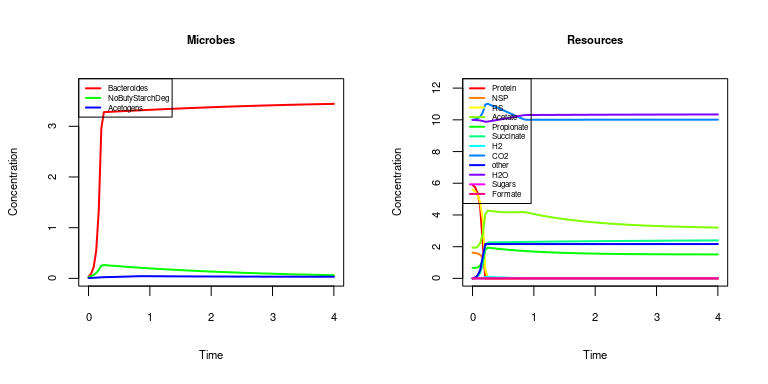### EXERCISE 1

Try adding in another included microbial dataset and see what happens. All 10 of the human colonic microbial groups have their information specified in the resourceSysInfoHuman and microbeSysInfoHuman data frames so that groups can be added or removed at will. Note, it is absolutely fine to have too much information in the SI files - microPop will just take the information needed for the microbes listed in microbeNames. [Hint: look at microbeSysInfoHuman to see the names of the microbes included in the human colon system.]

## Demofile: human2.R

Now we add in a pH change half way through the simulation time


simulation.times=seq(0,4,1/24)

myRateFuncs=rateFuncsDefault

myRateFuncs\$pHFunc=function(time,parms){
if (time<=max(simulation.times)/2){pH=5.5}else{pH=6.5}
return(pH)
}

out=microPopModel(
microbeNames=c('Bacteroides','NoButyStarchDeg','Acetogens'),
times=simulation.times,
resourceSysInfo=resourceSysInfoHuman,
microbeSysInfo=microbeSysInfoHuman,
rateFuncs=myRateFuncs,
pHLimit=TRUE,
plotOptions = list(plotFig=FALSE)
)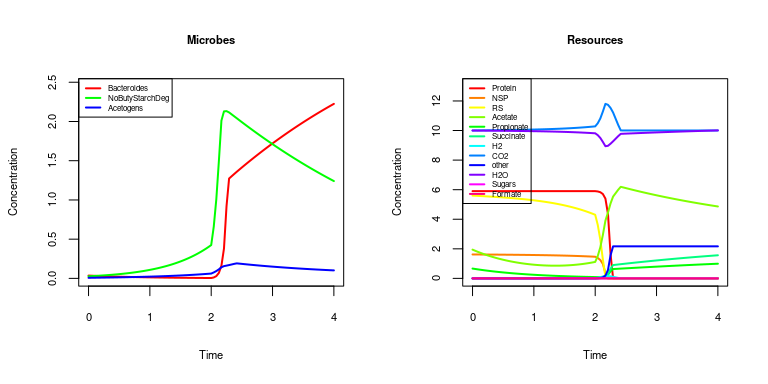## EXERCISE

Try changing the pHcorners for Bacteroides and look at what happens [Hint: copy Bacteroides to a new data frame e.g. myBacteroides and then edit that one and replace Bacteroides in microbeNames]

#SOLUTION

myBacteroides=Bacteroides

print(myBacteroides['pHcorners',])
#>           units Protein  NSP   RS Acetate Propionate Succinate H2 CO2 other
#> pHcorners    pH     5.6 6.35 7.85     8.6
print(myBacteroides['pHcorners',2:5])
#>           Protein  NSP   RS Acetate
#> pHcorners     5.6 6.35 7.85     8.6
myBacteroides['pHcorners',2:5]=c(5,6,7,8)

microbeHuman.SI = cbind(microbeSysInfoHuman,myBacteroides=microbeSysInfoHuman[,'Bacteroides']) #add Sys Info

out=microPopModel(
microbeNames=c('myBacteroides','NoButyStarchDeg','Acetogens'),
times=simulation.times,
resourceSysInfo=resourceSysInfoHuman,
microbeSysInfo=microbeHuman.SI,
rateFuncs=myRateFuncs,
pHLimit=TRUE
)
#>  "Set up completed, ODE solver called..."

# Stoichiometries and essential resources

In the M3 dataframe there are two substrates S1, S2 and two products P1, P2. The molar masses of these are defined in res.SI2 and are S1=100 g/mol, S2=100 g/mol, P1=50 g/mol and P2=25 g/mol. The stoichiometry will only balance if either S1 or S2 is used - not both.

2 S1 or 2 S2 -> 3 P1 +2 P2

2 x 100g or 2 x 100g -> 3 x 50g + 2 x 25g

This is because these are substitutable resources.

units S1 S2 P1 P2
Rtype none S S P P
halfSat g/l 0.001 0.001 NA NA
yield g/g 0.3 0.3 NA NA
maxGrowthRate /d 5 10 NA NA
stoichiom mol 2 2 3 2
keyResource none
pHcorners pH 4 5 7 8
numPathways none 1

The substrates are given Rtype S for substitutable, i.e. M3, can grow on either/both S1 and S2. This means that the stoichiom entry means 2 mol of S1 or 2 mol of S2 will give 3 mol of P1 and 2 mol of P2.

If both substrates were required for growth these would be given an Rtype of Se for essential substrate. E.g., methanogens require both CO2 and H2 to produce methane. Methanogens are an included data frame and can be viewed without loading. There is a version for the human colon (Methanogens) and for the rumen (Xh2). We will look at Xh2 as it is a simpler version (no formate path):

units Sh2 Biomass Sch4 SIC Snh3
Rtype none Se Pb P Se Se
halfSat g/l 0.00001168 NA NA 1E-22 0.0034
yield g/g 0.0904 NA NA NA NA
maxGrowthRate /h 0.0223 NA NA NA NA
stoichiom moles 1 0.0016 0.246 0.254 0.0016
keyResource none Sh2

Notice here that the microbial biomass is also included as a product in the stoichiometry (and therefore ammonia is also included as a substrate). The prefix 'S' indicates a soluble element.

When we are dealing with essential resources the keyResource category is used by microPop. This is needed as it is the substrate used for the yield value i.e. if H2 (Sh2) is the keyResource then a yield of 0.0904 is 0.09g of methanogens per 1 g of H2.

## Growth Equations

For growth on essential resources, all substrates must be present for growth therefore if we have two substrates S1 and S2, we use: $G=G^{\max} \frac{S1}{(S1+K1)}\frac{S2}{(S2+K2)}$ Whereas for substitutable resources, not all substrates need be present. However, if 2 substrates are available this does not mean the microbe will grow at twice the speed than if only one were present. There is a penalty for using two and this is is incorporated into the growth equations so that the specific growth rate on S1 if both S1 and S2 is given by: $G_1=G_{S1}^{\max}\frac{S1}{K1\left(1+\frac{S1}{K1}+\frac{S2}{K2}\right)}$ And the growth on S2 is given by $G_2=G_{S2}^{\max}\frac{S2}{K2\left(1+\frac{S1}{K1}+\frac{S2}{K2}\right)}$ We then use $$G = G_1 + G_2$$ to compute the total specific growth. Note that if only one substrate is present this reverts to simple Monod Equation growth. Also note that for substitutable resources the maximum growth rate on each resource can be different. For essential resources the microbe only has one maximum growth rate and this is specified to be on the keyResource.